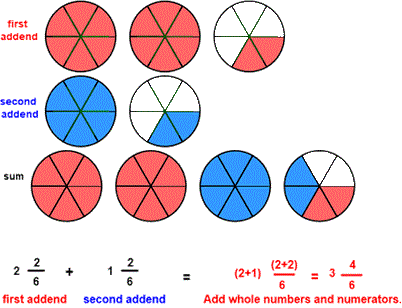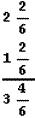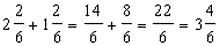CORRECT:
ATTEMPTS:
SCORE:
PERCENT
WHOLE
NUMERATOR
DENOMINATOR

# ADDITION OF LIKE FRACTIONS INSTRUCTIONS

Follow the directions in the dialog box after pressing the <START> button. The <EXPLAIN> button may be pressed after you enter the second addend to see how to do the example.

When the program starts, you will be asked to identify the first addend and then the second addend. The program will not continue unless each addend is correctly identified. You will then be asked to add each addend for the sum.

The following picture was made by:You can see from the picture that the first addend is 2 complete units and 26 partial unit in length and the second addend is 1 complete unit and 26 partial unit in length. The sum will combine the complete units for 3 complete units and the partial units for 46 partial units for a sum of 3 46 units. The sum in the picture shows the first addend in red combined with the second addend in blue for a sum of 3 46.

To calculate the Sum first add the whole numbers 2 and 1 to obtain 3 for a whole number. Then add the numerators of the fractions 26 and 26 to obtain the 46 in the sum. You may enter 3 46 or the simplified form 3 23

You may prefer to work vertically:You may prefer to write each addend in fraction form first as in this example:You may enter the sum in fraction form or mixed number form. In the above example 226 or 3 46 are both acceptable.

For more instruction on adding fractions go to How To Add Fractions.

After you enter the sum you may press the <REPORT> button. The report will ask for your name but you may submit a code for your name. This report will give the same results as on the dialog box. The report may be printed or e-mailed.